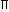# Aptitude - Volume and Surface Area - Discussion

Discussion Forum : Volume and Surface Area - Data Sufficiency 1 (Q.No. 3)
Directions to Solve

Each of the questions given below consists of a statement and / or a question and two statements numbered I and II given below it. You have to decide whether the data provided in the statement(s) is / are sufficient to answer the given question. Read the both statements and

• Give answer (A) if the data in Statement I alone are sufficient to answer the question, while the data in Statement II alone are not sufficient to answer the question.
• Give answer (B) if the data in Statement II alone are sufficient to answer the question, while the data in Statement I alone are not sufficient to answer the question.
• Give answer (C) if the data either in Statement I or in Statement II alone are sufficient to answer the question.
• Give answer (D) if the data even in both Statements I and II together are not sufficient to answer the question.
• Give answer(E) if the data in both Statements I and II together are necessary to answer the question.

3.
 What is the capacity of a cylindrical tank? I. Radius of the base is half of its height which is 28 metres. II. Area of the base is 616 sq. metres and its height is 28 metres.
I alone sufficient while II alone not sufficient to answer
II alone sufficient while I alone not sufficient to answer
Either I or II alone sufficient to answer
Both I and II are not sufficient to answer
Both I and II are necessary to answer
Explanation:

I gives, h = 28 m and r = 14.Capacity =r2h, which can be obtained.

Thus, I alone gives the answer.

II gives,r2 = 616 m2 and h = 28 m.Capacity = (r2 x h) = (616 x 28) m3.

Thus, II alone gives the answer.Correct answer is (C).

Discussion:
1 comments Page 1 of 1.